• Slides: 83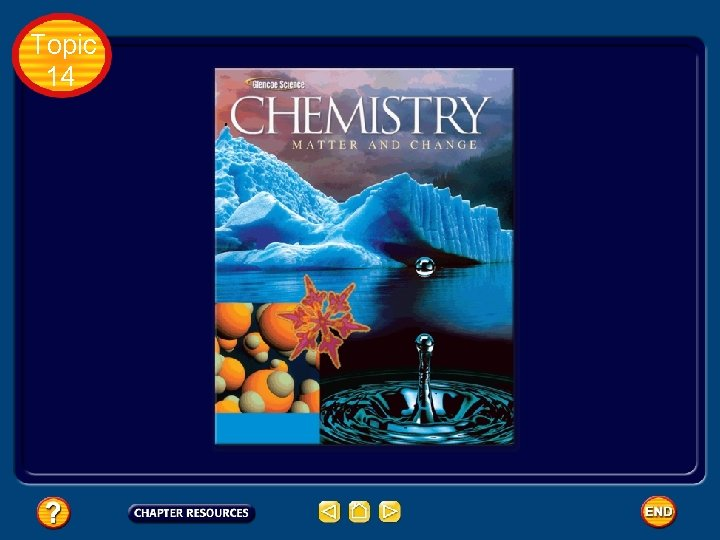Topic 14Topic 14 The Mole: Basic Concepts Measuring Matter • Chemists need a convenient method for counting accurately the number of atoms, molecules, or formula units in a sample of a substance. • As you know, atoms and molecules are extremely small. There are so many of them in even the smallest sample that it’s impossible to actually count them. • That’s why chemists created their own counting unit called the mole.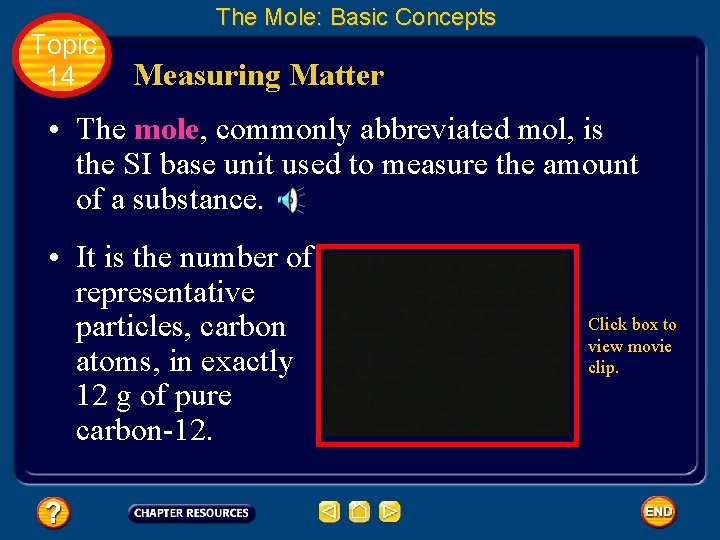Topic 14 The Mole: Basic Concepts Measuring Matter • The mole, commonly abbreviated mol, is the SI base unit used to measure the amount of a substance. • It is the number of representative particles, carbon atoms, in exactly 12 g of pure carbon-12. Click box to view movie clip.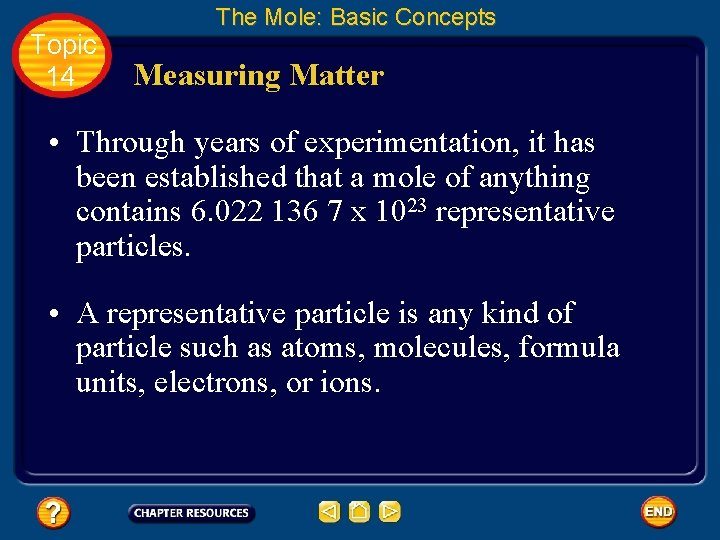Topic 14 The Mole: Basic Concepts Measuring Matter • Through years of experimentation, it has been established that a mole of anything contains 6. 022 136 7 x 1023 representative particles. • A representative particle is any kind of particle such as atoms, molecules, formula units, electrons, or ions.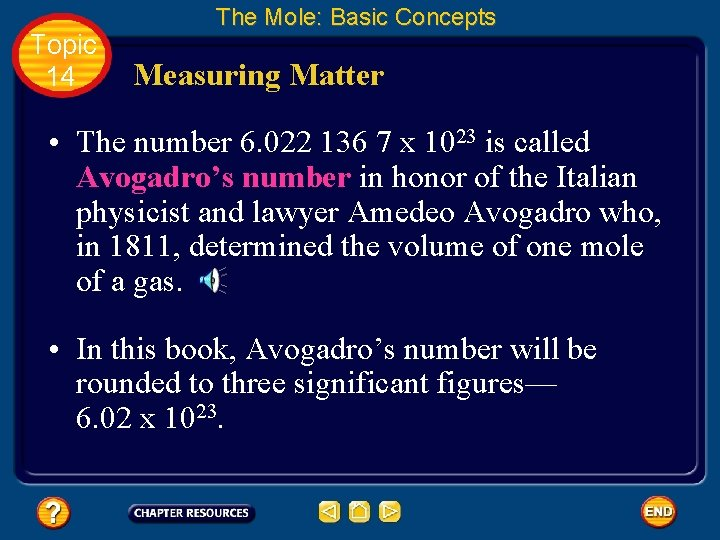Topic 14 The Mole: Basic Concepts Measuring Matter • The number 6. 022 136 7 x 1023 is called Avogadro’s number in honor of the Italian physicist and lawyer Amedeo Avogadro who, in 1811, determined the volume of one mole of a gas. • In this book, Avogadro’s number will be rounded to three significant figures— 6. 02 x 1023.Topic 14 The Mole: Basic Concepts Measuring Matter • If you write out Avogadro’s number, it looks like this. 602 000 000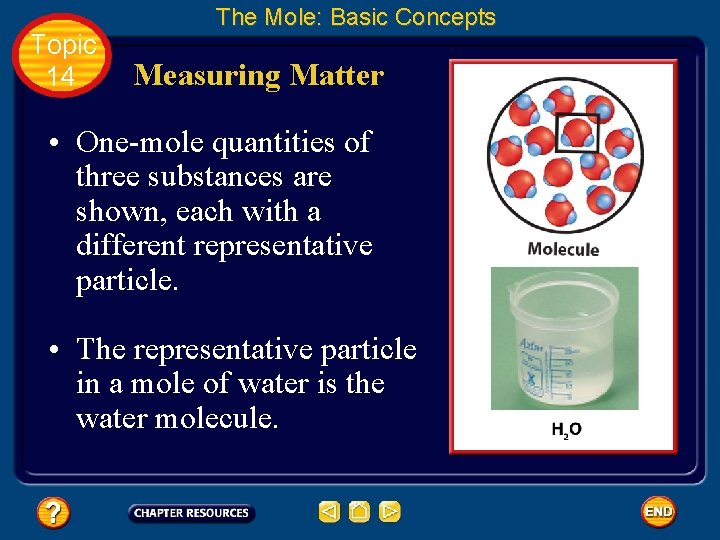Topic 14 The Mole: Basic Concepts Measuring Matter • One-mole quantities of three substances are shown, each with a different representative particle. • The representative particle in a mole of water is the water molecule.Topic 14 The Mole: Basic Concepts Measuring Matter • The representative particle in a mole of copper is the copper atom.Topic 14 The Mole: Basic Concepts Measuring Matter • The representative particle in a mole of sodium chloride is the formula unit.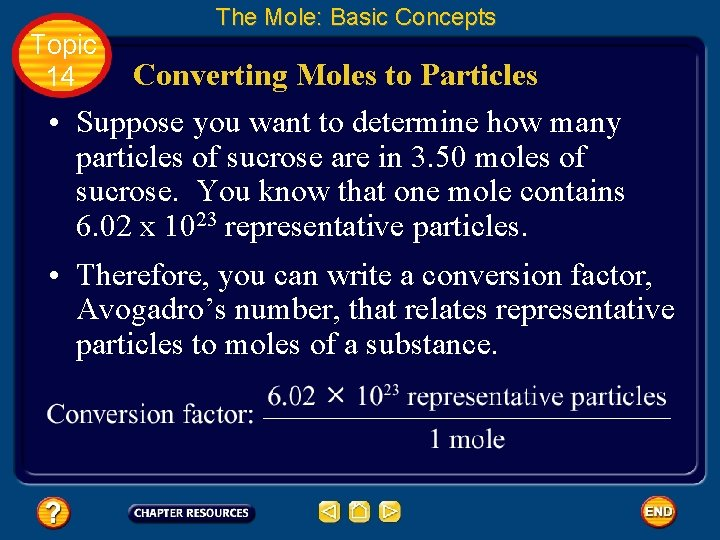Topic 14 The Mole: Basic Concepts Converting Moles to Particles • Suppose you want to determine how many particles of sucrose are in 3. 50 moles of sucrose. You know that one mole contains 6. 02 x 1023 representative particles. • Therefore, you can write a conversion factor, Avogadro’s number, that relates representative particles to moles of a substance.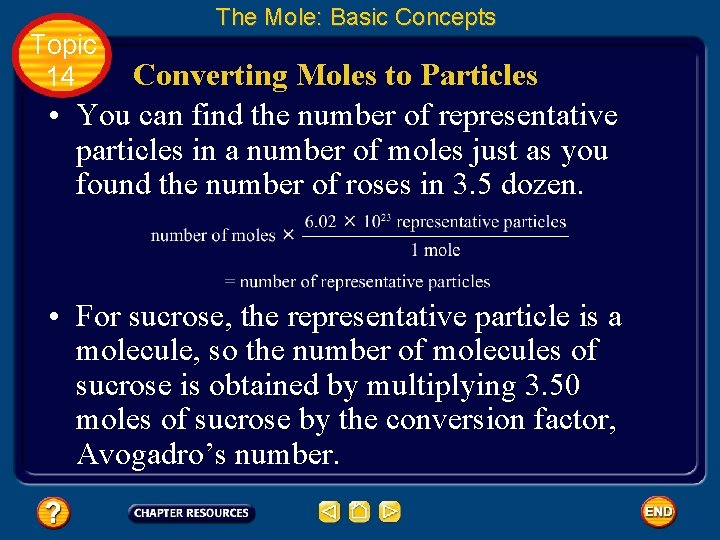Topic 14 The Mole: Basic Concepts Converting Moles to Particles • You can find the number of representative particles in a number of moles just as you found the number of roses in 3. 5 dozen. • For sucrose, the representative particle is a molecule, so the number of molecules of sucrose is obtained by multiplying 3. 50 moles of sucrose by the conversion factor, Avogadro’s number.Topic 14 The Mole: Basic Concepts Converting Moles to Particles • There are 2. 11 x 1024 molecules of sucrose in 3. 50 moles.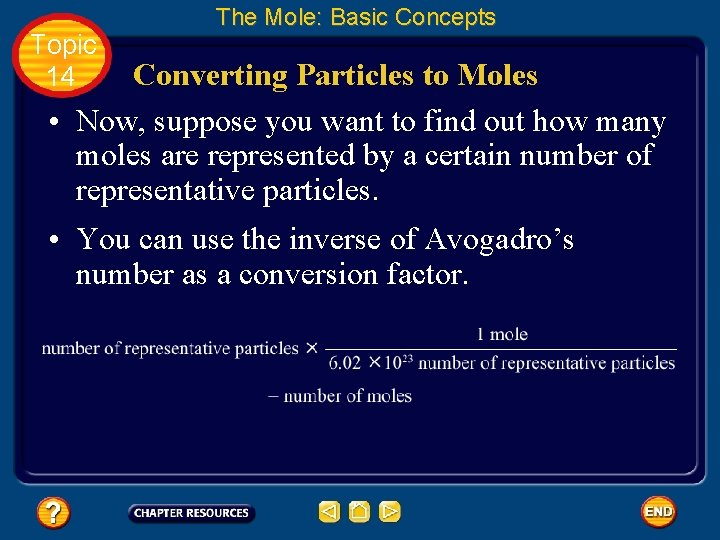Topic 14 The Mole: Basic Concepts Converting Particles to Moles • Now, suppose you want to find out how many moles are represented by a certain number of representative particles. • You can use the inverse of Avogadro’s number as a conversion factor.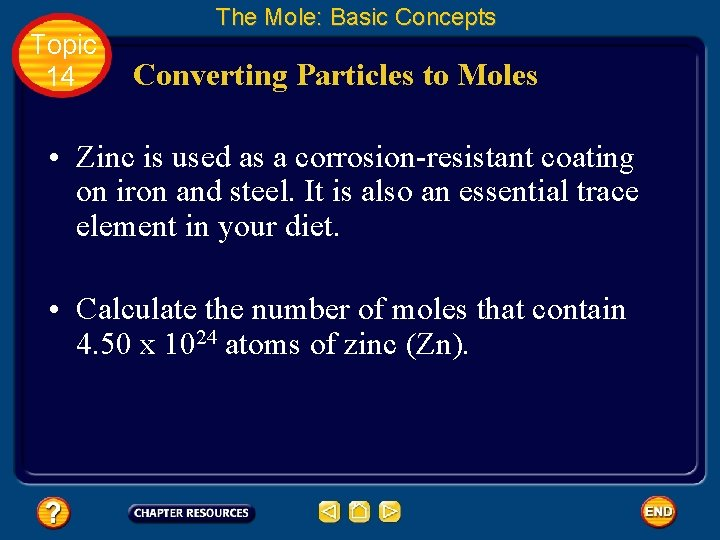Topic 14 The Mole: Basic Concepts Converting Particles to Moles • Zinc is used as a corrosion-resistant coating on iron and steel. It is also an essential trace element in your diet. • Calculate the number of moles that contain 4. 50 x 1024 atoms of zinc (Zn).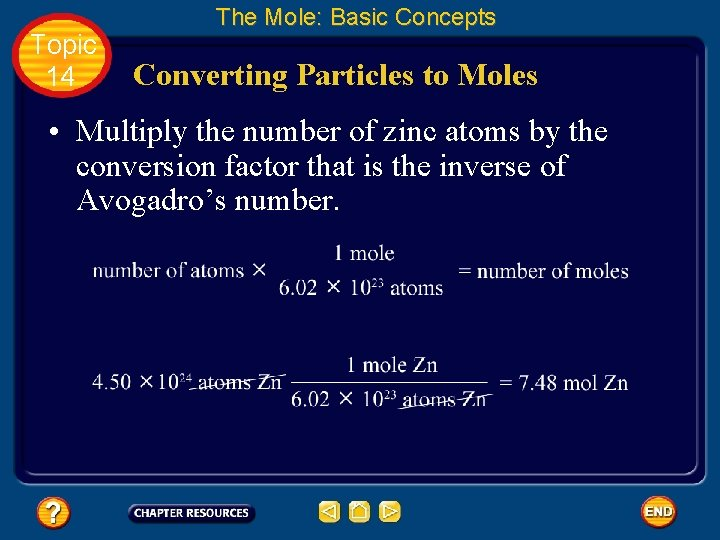Topic 14 The Mole: Basic Concepts Converting Particles to Moles • Multiply the number of zinc atoms by the conversion factor that is the inverse of Avogadro’s number.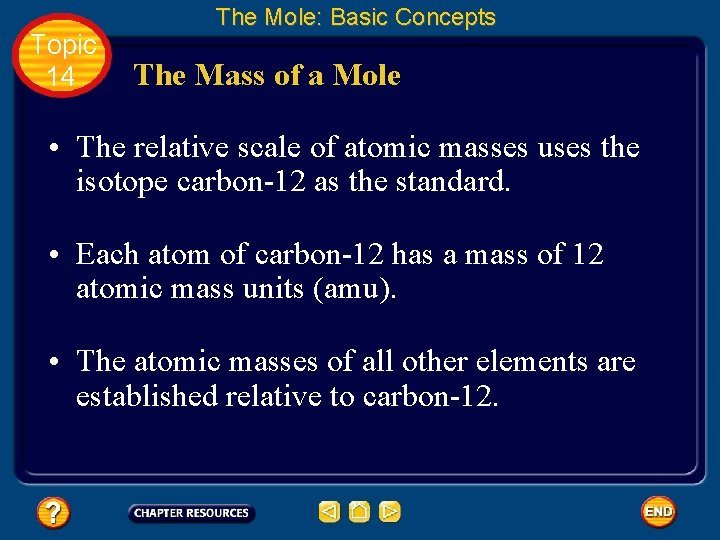Topic 14 The Mole: Basic Concepts The Mass of a Mole • The relative scale of atomic masses uses the isotope carbon-12 as the standard. • Each atom of carbon-12 has a mass of 12 atomic mass units (amu). • The atomic masses of all other elements are established relative to carbon-12.Topic 14 The Mole: Basic Concepts The Mass of a Mole • For example, an atom of hydrogen-1 has a mass of 1 amu. • The mass of an atom of helium-4 is 4 amu. • Therefore, the mass of one atom of hydrogen-1 is one-twelfth the mass of one atom of carbon-12. • The mass of one atom of helium-4 is onethird the mass of one atom of carbon-12.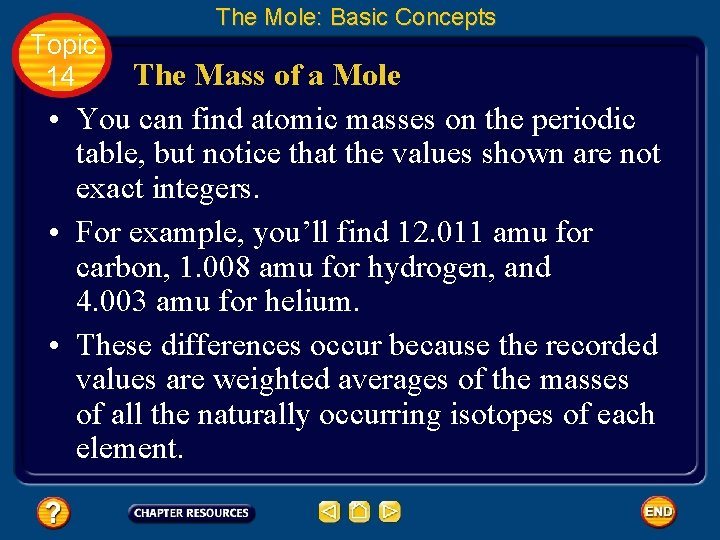Topic 14 The Mole: Basic Concepts The Mass of a Mole • You can find atomic masses on the periodic table, but notice that the values shown are not exact integers. • For example, you’ll find 12. 011 amu for carbon, 1. 008 amu for hydrogen, and 4. 003 amu for helium. • These differences occur because the recorded values are weighted averages of the masses of all the naturally occurring isotopes of each element.Topic 14 The Mole: Basic Concepts The Mass of a Mole • You know that the mole is defined as the number of representative particles, or carbon 12 atoms, in exactly 12 g of pure carbon-12. • Thus, the mass of one mole of carbon-12 atoms is 12 g. What about other elements? • Whether you are considering a single atom or Avogadro’s number of atoms (a mole), the masses of all atoms are established relative to the mass of carbon-12.Topic 14 The Mole: Basic Concepts The Mass of a Mole • The mass of a mole of hydrogen-1 is onetwelfth the mass of a mole of carbon-12 atoms, or 1. 0 g. • The mass of a mole of helium-4 atoms is one -third the mass of a mole of carbon-12 atoms, or 4. 0 g.Topic 14 The Mole: Basic Concepts The Mass of a Mole • The mass in grams of one mole of any pure substance is called its molar mass. • The molar mass of any element is numerically equal to its atomic mass and has the units g/mol.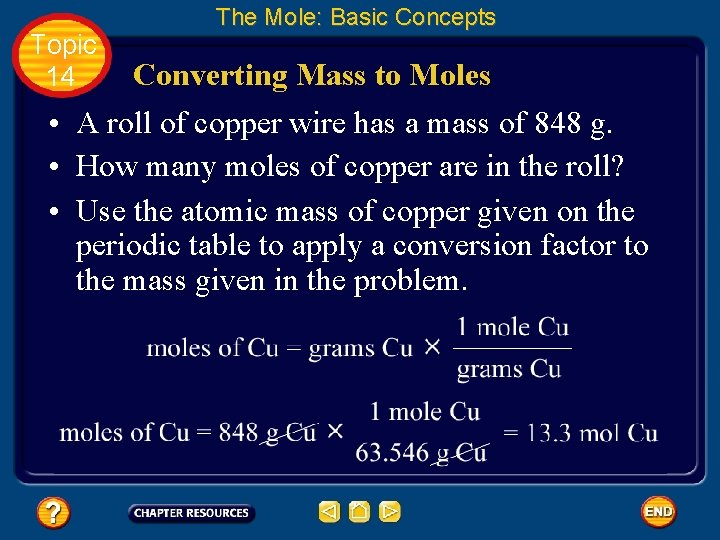Topic 14 The Mole: Basic Concepts Converting Mass to Moles • A roll of copper wire has a mass of 848 g. • How many moles of copper are in the roll? • Use the atomic mass of copper given on the periodic table to apply a conversion factor to the mass given in the problem.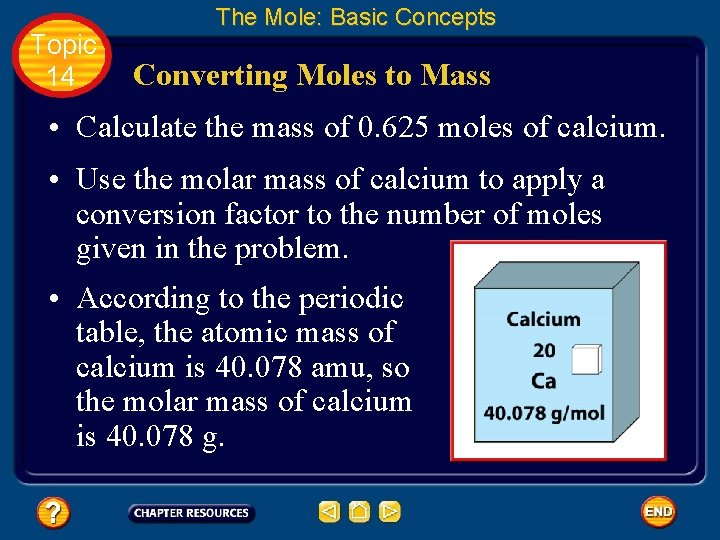Topic 14 The Mole: Basic Concepts Converting Moles to Mass • Calculate the mass of 0. 625 moles of calcium. • Use the molar mass of calcium to apply a conversion factor to the number of moles given in the problem. • According to the periodic table, the atomic mass of calcium is 40. 078 amu, so the molar mass of calcium is 40. 078 g.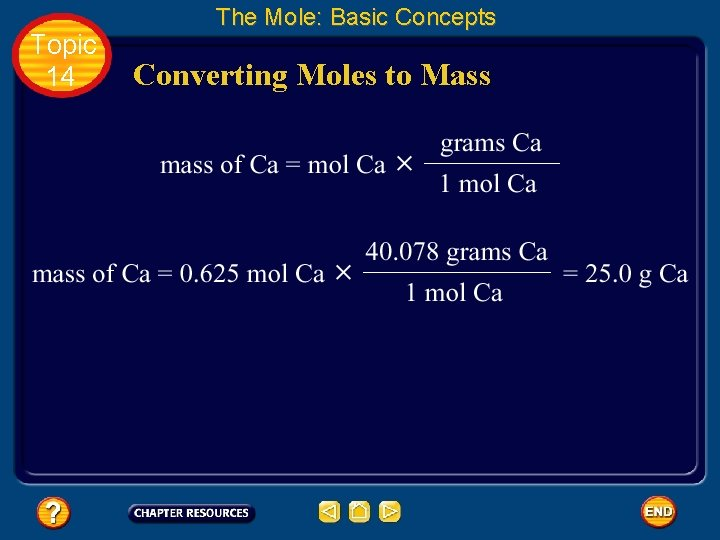Topic 14 The Mole: Basic Concepts Converting Moles to Mass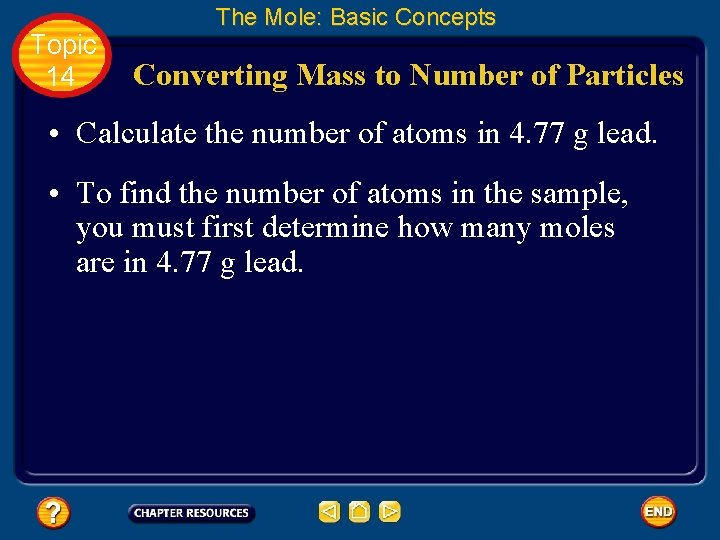Topic 14 The Mole: Basic Concepts Converting Mass to Number of Particles • Calculate the number of atoms in 4. 77 g lead. • To find the number of atoms in the sample, you must first determine how many moles are in 4. 77 g lead.Topic 14 The Mole: Basic Concepts Converting Mass to Number of Particles • According to data from the periodic table, the molar mass of lead is 207. 2 g/mol. Apply a conversion factor to convert mass to moles.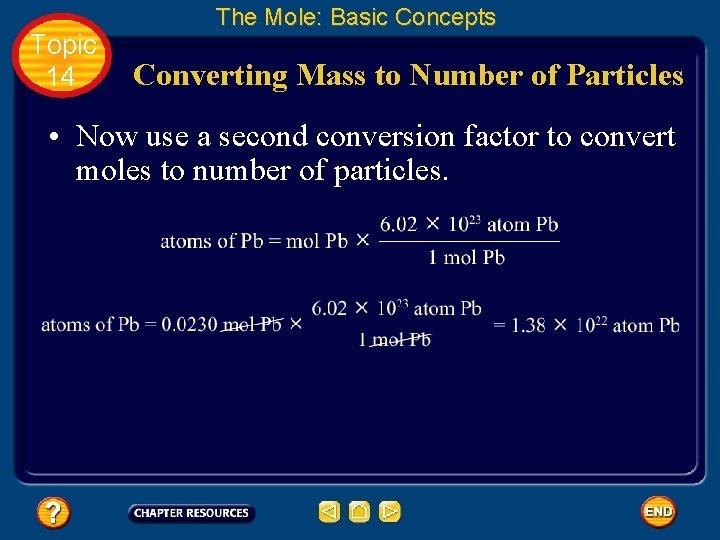Topic 14 The Mole: Basic Concepts Converting Mass to Number of Particles • Now use a second conversion factor to convert moles to number of particles.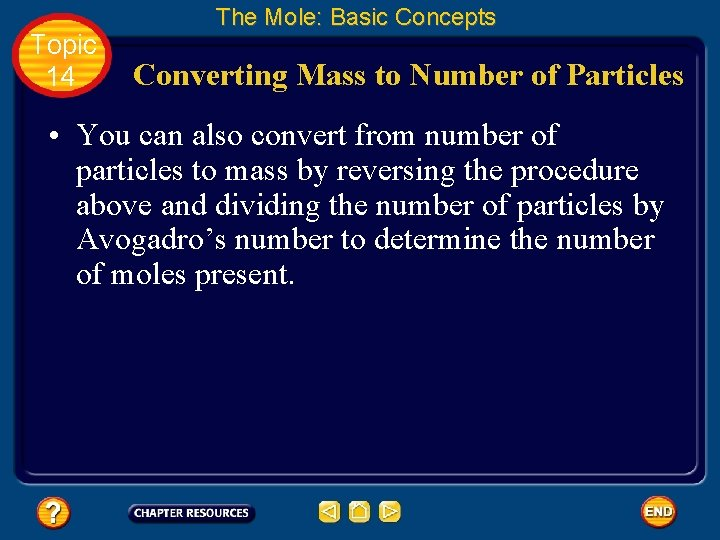Topic 14 The Mole: Basic Concepts Converting Mass to Number of Particles • You can also convert from number of particles to mass by reversing the procedure above and dividing the number of particles by Avogadro’s number to determine the number of moles present.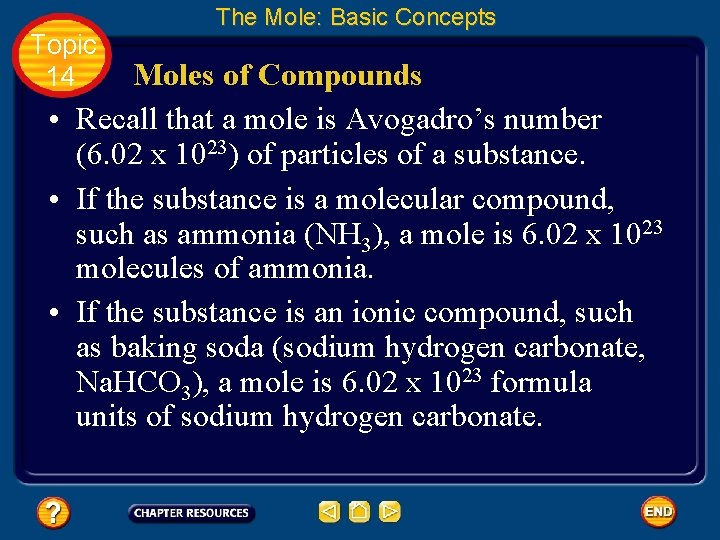Topic 14 The Mole: Basic Concepts Moles of Compounds • Recall that a mole is Avogadro’s number (6. 02 x 1023) of particles of a substance. • If the substance is a molecular compound, such as ammonia (NH 3), a mole is 6. 02 x 1023 molecules of ammonia. • If the substance is an ionic compound, such as baking soda (sodium hydrogen carbonate, Na. HCO 3), a mole is 6. 02 x 1023 formula units of sodium hydrogen carbonate.Topic 14 The Mole: Basic Concepts Moles of Compounds • In either case, a mole of a compound contains as many moles of each element as are indicated by the subscripts in the formula for the compound. • For example, a mole of ammonia (NH 3) consists of one mole of nitrogen atoms and three moles of hydrogen atoms.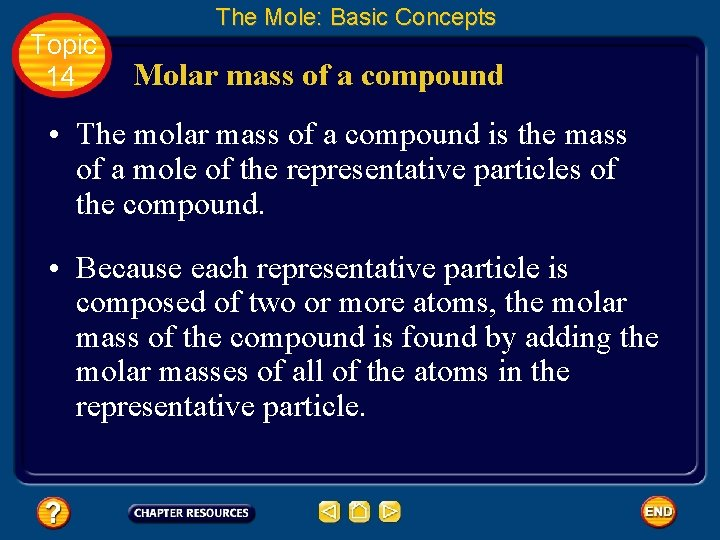Topic 14 The Mole: Basic Concepts Molar mass of a compound • The molar mass of a compound is the mass of a mole of the representative particles of the compound. • Because each representative particle is composed of two or more atoms, the molar mass of the compound is found by adding the molar masses of all of the atoms in the representative particle.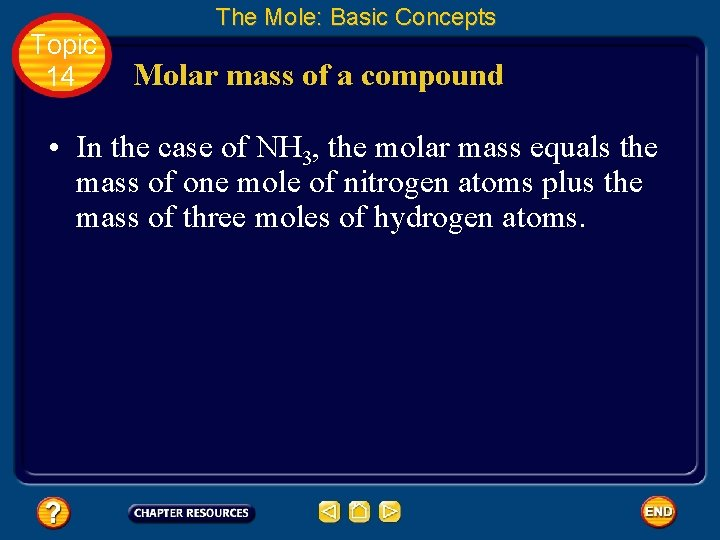Topic 14 The Mole: Basic Concepts Molar mass of a compound • In the case of NH 3, the molar mass equals the mass of one mole of nitrogen atoms plus the mass of three moles of hydrogen atoms.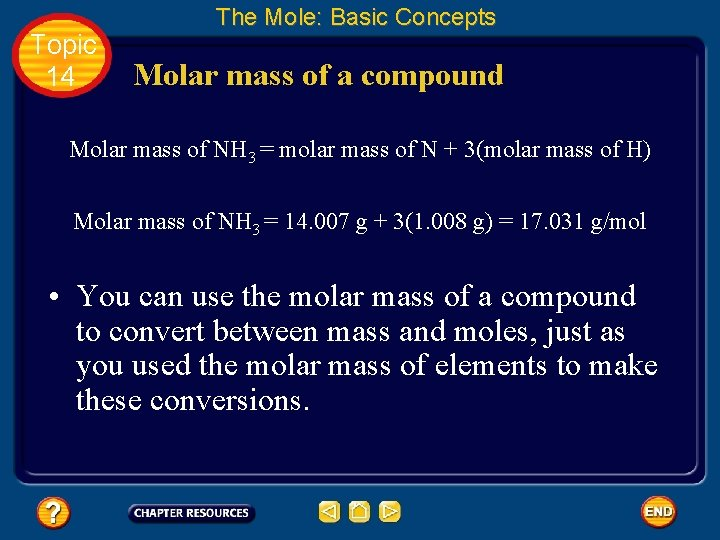Topic 14 The Mole: Basic Concepts Molar mass of a compound Molar mass of NH 3 = molar mass of N + 3(molar mass of H) Molar mass of NH 3 = 14. 007 g + 3(1. 008 g) = 17. 031 g/mol • You can use the molar mass of a compound to convert between mass and moles, just as you used the molar mass of elements to make these conversions.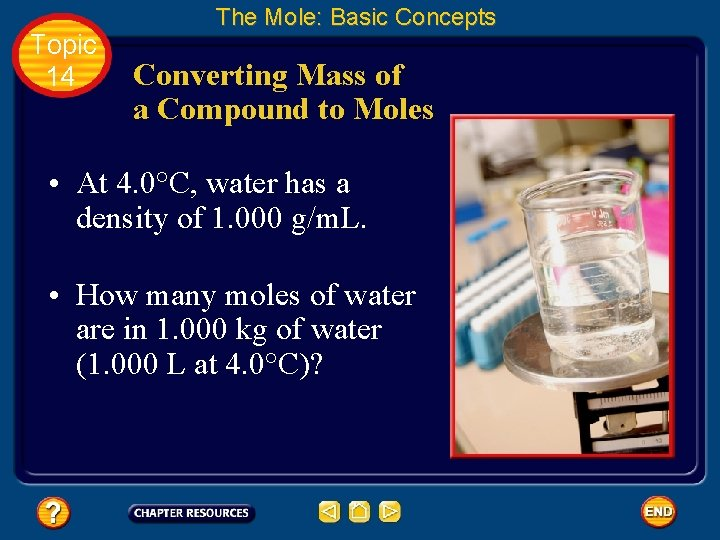Topic 14 The Mole: Basic Concepts Converting Mass of a Compound to Moles • At 4. 0°C, water has a density of 1. 000 g/m. L. • How many moles of water are in 1. 000 kg of water (1. 000 L at 4. 0°C)?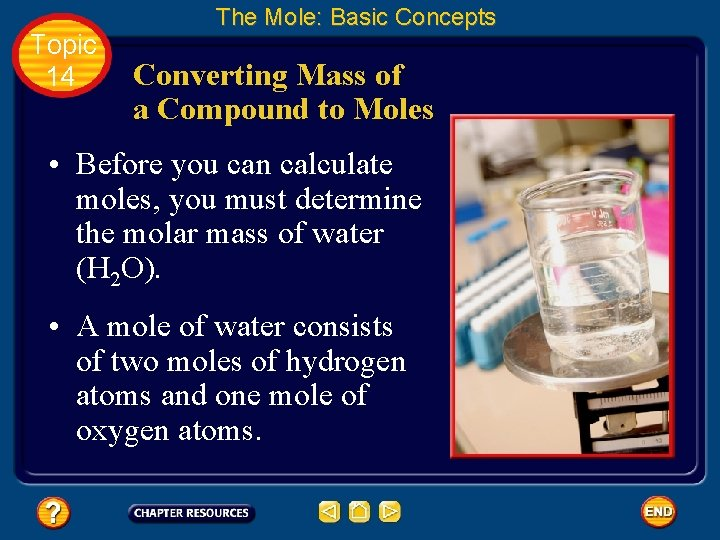Topic 14 The Mole: Basic Concepts Converting Mass of a Compound to Moles • Before you can calculate moles, you must determine the molar mass of water (H 2 O). • A mole of water consists of two moles of hydrogen atoms and one mole of oxygen atoms.Topic 14 The Mole: Basic Concepts Converting Mass of a Compound to Moles molar mass H 2 O = 2(molar mass H) + molar mass O • Now you can use the molar mass of water as a conversion factor to determine moles of water. • Notice that 1. 000 kg is converted to 1. 000 x 103 g for the calculation.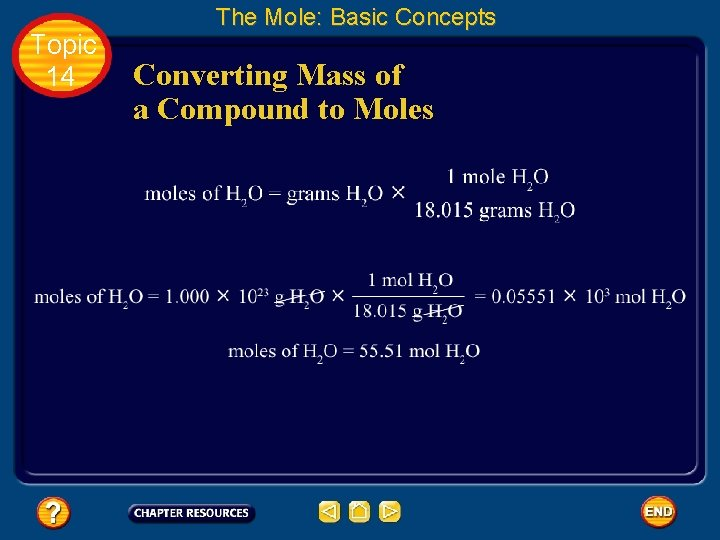Topic 14 The Mole: Basic Concepts Converting Mass of a Compound to MolesTopic 14 Basic Assessment Questions Question 1 Calculate the number of molecules in 15. 7 mol carbon dioxide.Topic 14 Basic Assessment Questions Answer 9. 45 x 1024 molecular CO 2Topic 14 Basic Assessment Questions Question 2 Calculate the number of moles in 9. 22 x 1023 atom iron.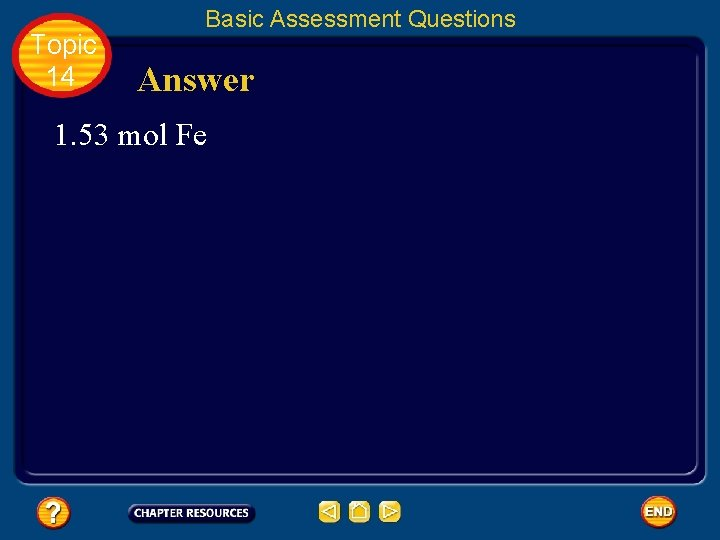Topic 14 Basic Assessment Questions Answer 1. 53 mol FeTopic 14 Basic Assessment Questions Question 3 Calculate the mass of 6. 89 mol antimony.Topic 14 Basic Assessment Questions Answer 839 g SbTopic 14 Basic Assessment Questions Question 4 A chemist needs 0. 0700 mol selenium for a reaction. What mass of selenium should the chemist use?Topic 14 Basic Assessment Questions Answer 5. 53 g Se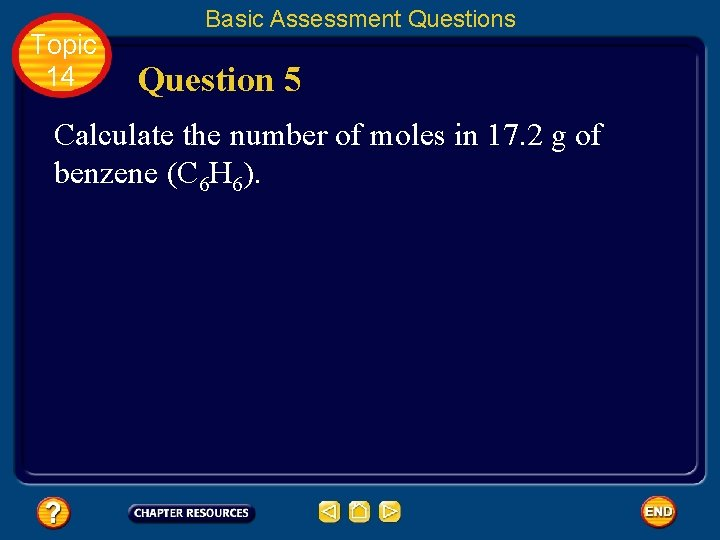Topic 14 Basic Assessment Questions Question 5 Calculate the number of moles in 17. 2 g of benzene (C 6 H 6).Topic 14 Basic Assessment Questions Answer 0. 220 mol C 6 H 6Topic 14 The Mole: Additional ConceptsTopic 14 The Mole: Additional Concepts Empirical and Molecular Formulas • Recall that every chemical compound has a definite composition—a composition that is always the same wherever that compound is found. • The composition of a compound is usually stated as the percent by mass of each element in the compound.Topic 14 The Mole: Additional Concepts Percent composition • The percent of an element in a compound can be found in the following way. Click box to view movie clip.Topic 14 The Mole: Additional Concepts Calculating Percent Composition • Determine the percent composition of calcium chloride (Ca. Cl 2). • First, analyze the information available from the formula. • A mole of calcium chloride consists of one mole of calcium ions and two moles of chloride ions.Topic 14 The Mole: Additional Concepts Calculating Percent Composition • Next, gather molar mass information from the atomic masses on the periodic table. • To the mass of one mole of Ca. Cl 2, a mole of calcium ions contributes 40. 078 g, and two moles of chloride ions contribute 2 x 35. 453 g = 70. 906 g for a total molar mass of 110. 984 g/mol for Ca. Cl 2.Topic 14 The Mole: Additional Concepts Calculating Percent Composition • Finally, use the data to set up a calculation to determine the percent by mass of each element in the compound. • The percent by mass of calcium and chlorine in Ca. Cl 2 can be calculated as follows.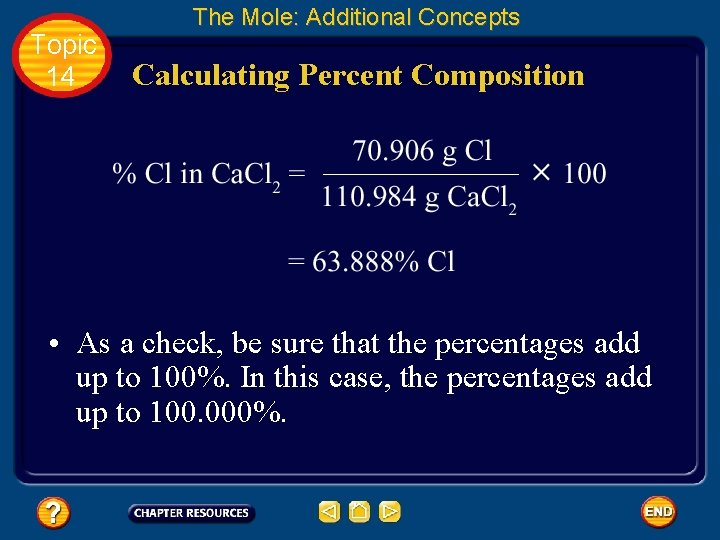Topic 14 The Mole: Additional Concepts Calculating Percent Composition • As a check, be sure that the percentages add up to 100%. In this case, the percentages add up to 100. 000%.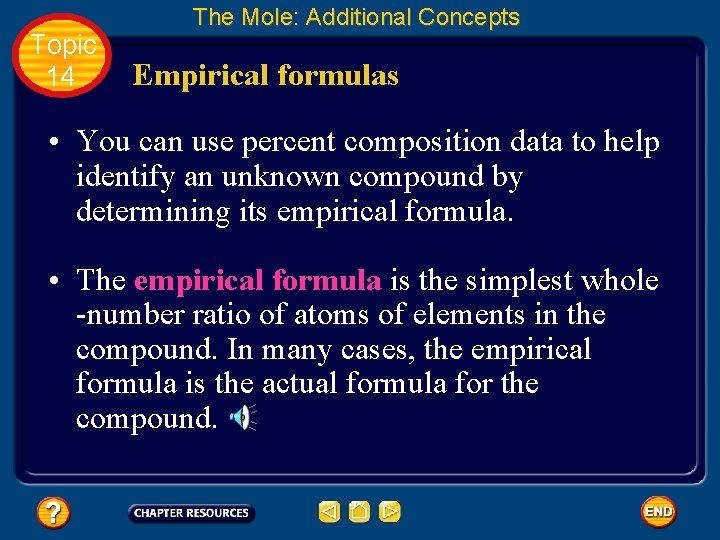Topic 14 The Mole: Additional Concepts Empirical formulas • You can use percent composition data to help identify an unknown compound by determining its empirical formula. • The empirical formula is the simplest whole -number ratio of atoms of elements in the compound. In many cases, the empirical formula is the actual formula for the compound.Topic 14 The Mole: Additional Concepts Empirical formulas • For example, the simplest ratio of atoms of sodium to atoms of chlorine in sodium chloride is 1 atom Na : 1 atom Cl. • So, the empirical formula of sodium chloride is Na 1 Cl 1, or Na. Cl, which is the true formula for the compound.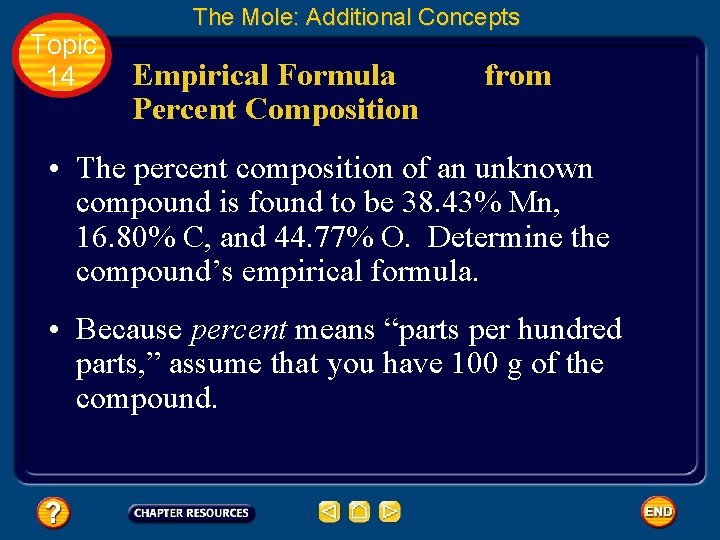Topic 14 The Mole: Additional Concepts Empirical Formula Percent Composition from • The percent composition of an unknown compound is found to be 38. 43% Mn, 16. 80% C, and 44. 77% O. Determine the compound’s empirical formula. • Because percent means “parts per hundred parts, ” assume that you have 100 g of the compound.Topic 14 The Mole: Additional Concepts Empirical Formula from Percent Composition • Then calculate the number of moles of each element in the 100 g of compound. • The number of moles of manganese may be calculated as follows.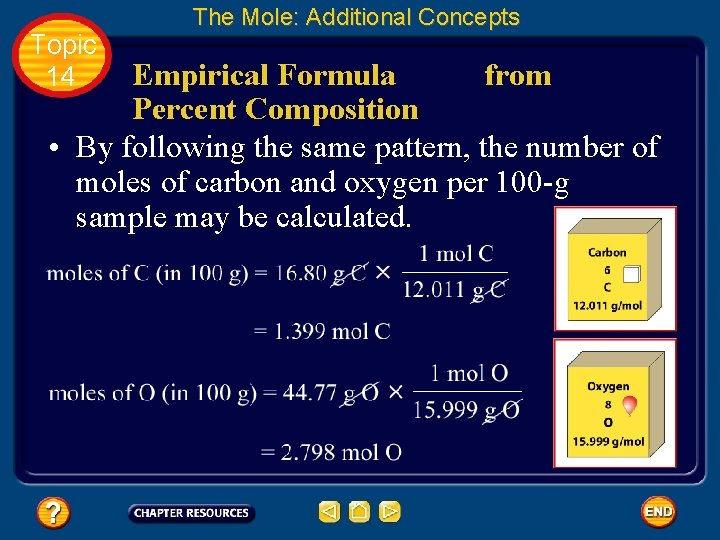Topic 14 The Mole: Additional Concepts Empirical Formula from Percent Composition • By following the same pattern, the number of moles of carbon and oxygen per 100 -g sample may be calculated.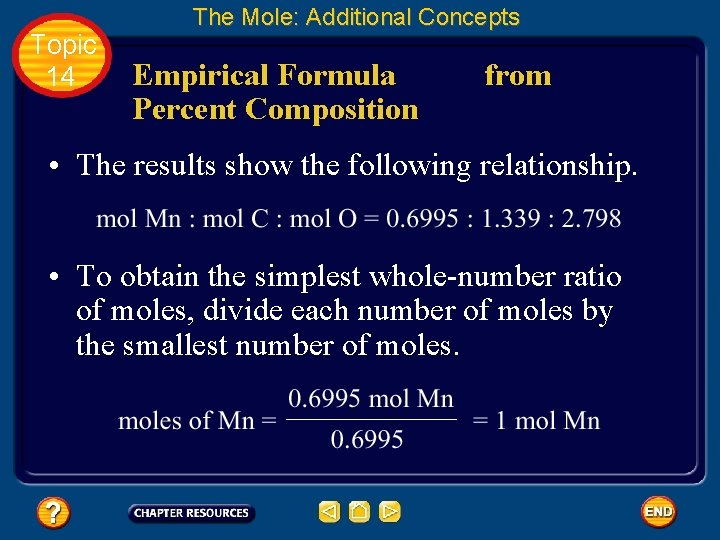Topic 14 The Mole: Additional Concepts Empirical Formula Percent Composition from • The results show the following relationship. • To obtain the simplest whole-number ratio of moles, divide each number of moles by the smallest number of moles.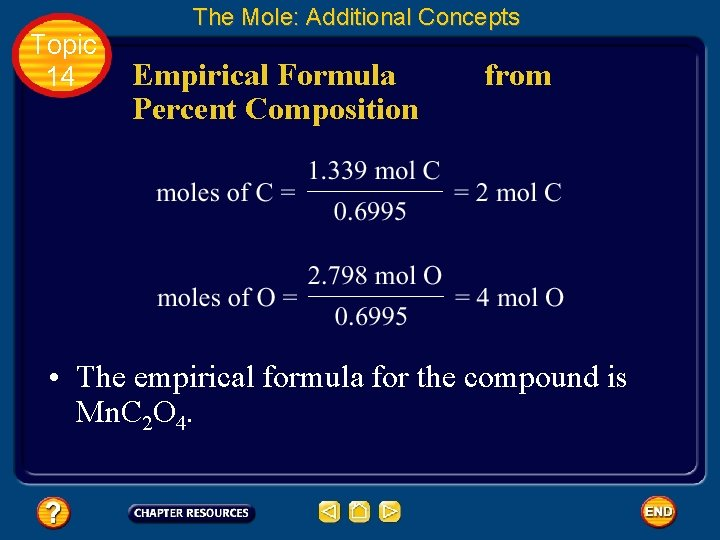Topic 14 The Mole: Additional Concepts Empirical Formula Percent Composition from • The empirical formula for the compound is Mn. C 2 O 4.Topic 14 The Mole: Additional Concepts Molecular formulas • For many compounds, the empirical formula is not the true formula. • Chemists have learned, though, that acetic acid is a molecule with the formula C 2 H 4 O 2, which is the molecular formula for acetic acid. • A molecular formula tells the exact number of atoms of each element in a molecule or formula unit of a compound.Topic 14 The Mole: Additional Concepts Molecular formulas • Notice that the molecular formula for acetic acid (C 2 H 4 O 2) has exactly twice as many atoms of each element as the empirical formula (CH 2 O). • The molecular formula for a compound is always a whole-number multiple of the empirical formula.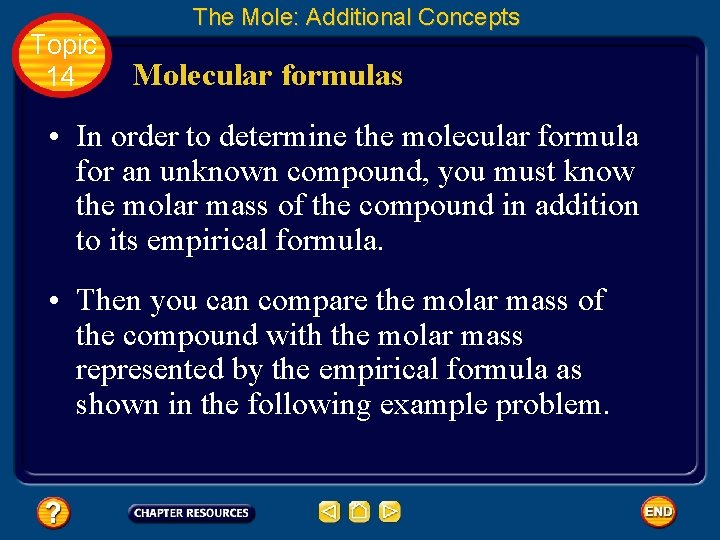Topic 14 The Mole: Additional Concepts Molecular formulas • In order to determine the molecular formula for an unknown compound, you must know the molar mass of the compound in addition to its empirical formula. • Then you can compare the molar mass of the compound with the molar mass represented by the empirical formula as shown in the following example problem.Topic 14 The Mole: Additional Concepts Determining a Molecular Formula • Maleic acid is a compound that is widely used in the plastics and textiles industries. • The composition of maleic acid is 41. 39% carbon, 3. 47% hydrogen, and 55. 14% oxygen. • Its molar mass is 116. 1 g/mol. • Calculate the molecular formula for maleic acid.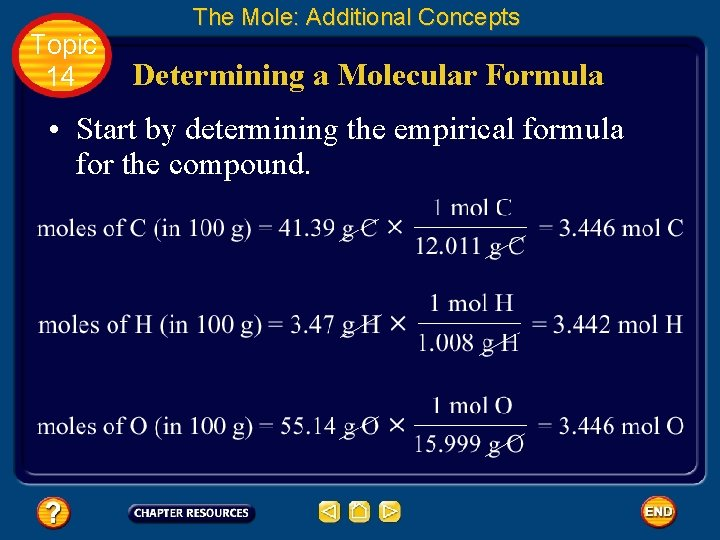Topic 14 The Mole: Additional Concepts Determining a Molecular Formula • Start by determining the empirical formula for the compound.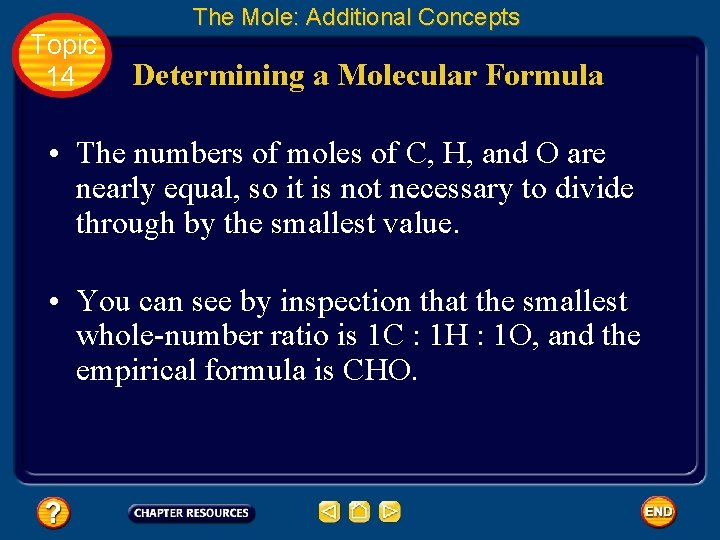Topic 14 The Mole: Additional Concepts Determining a Molecular Formula • The numbers of moles of C, H, and O are nearly equal, so it is not necessary to divide through by the smallest value. • You can see by inspection that the smallest whole-number ratio is 1 C : 1 H : 1 O, and the empirical formula is CHO.Topic 14 The Mole: Additional Concepts Determining a Molecular Formula • Next, calculate the molar mass represented by the formula CHO. • Here, the molar mass is the sum of the masses of one mole of each element.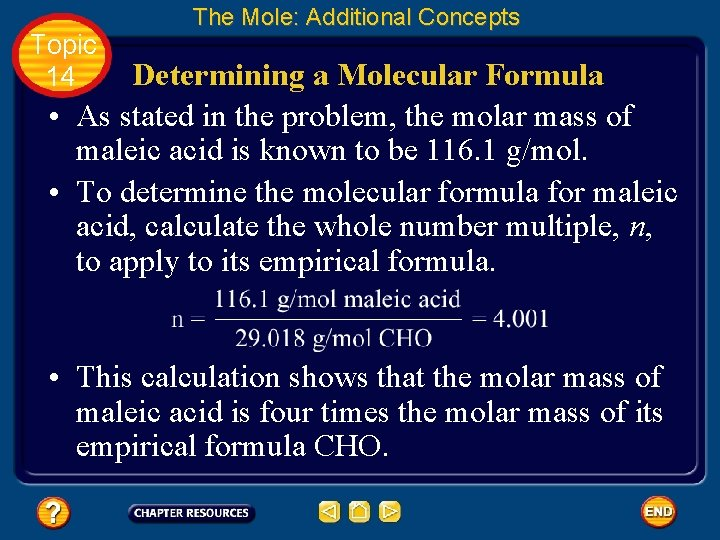Topic 14 The Mole: Additional Concepts Determining a Molecular Formula • As stated in the problem, the molar mass of maleic acid is known to be 116. 1 g/mol. • To determine the molecular formula for maleic acid, calculate the whole number multiple, n, to apply to its empirical formula. • This calculation shows that the molar mass of maleic acid is four times the molar mass of its empirical formula CHO.Topic 14 The Mole: Additional Concepts Determining a Molecular Formula • Therefore, the molecular formula must have four times as many atoms of each element as the empirical formula. • Thus, the molecular formula is (CHO)4 = C 4 H 4 O 4 • A check of the molecular formula for maleic acid in a reference book will confirm this result.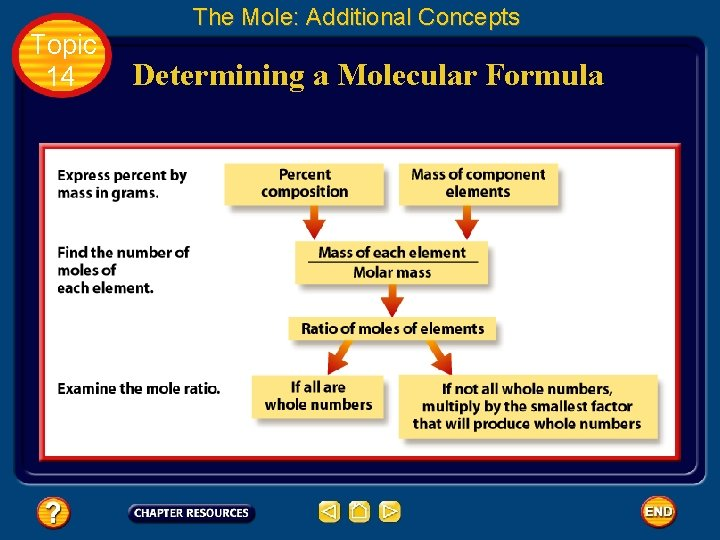Topic 14 The Mole: Additional Concepts Determining a Molecular FormulaTopic 14 The Mole: Additional Concepts Determining a Molecular Formula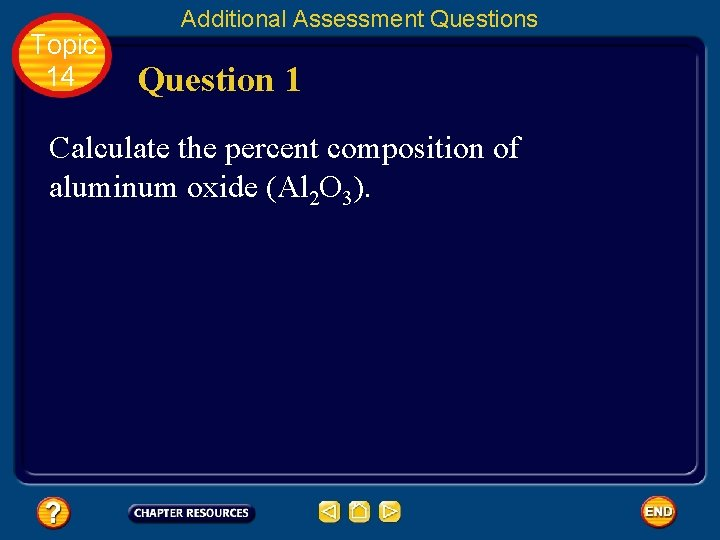Topic 14 Additional Assessment Questions Question 1 Calculate the percent composition of aluminum oxide (Al 2 O 3).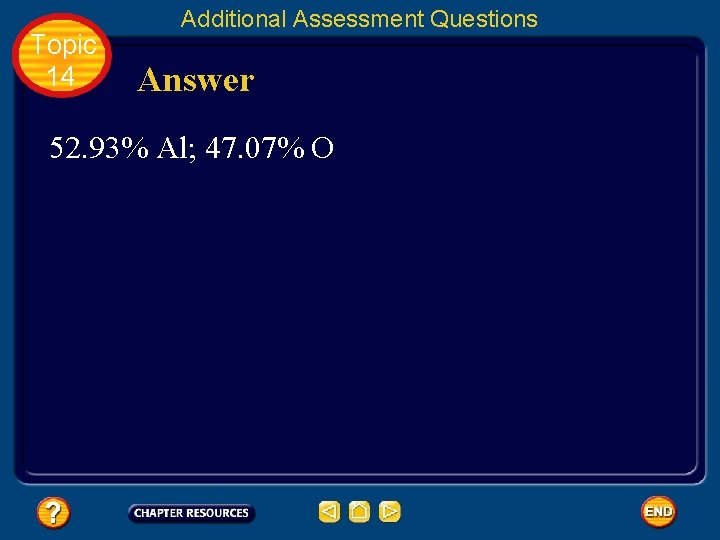Topic 14 Additional Assessment Questions Answer 52. 93% Al; 47. 07% O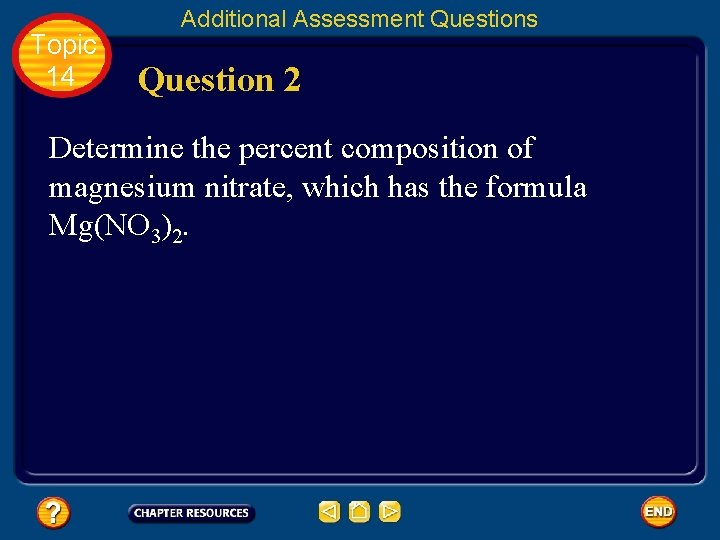Topic 14 Additional Assessment Questions Question 2 Determine the percent composition of magnesium nitrate, which has the formula Mg(NO 3)2.Topic 14 Additional Assessment Questions Answer 16. 39% Mg; 18. 89% N; 64. 72% O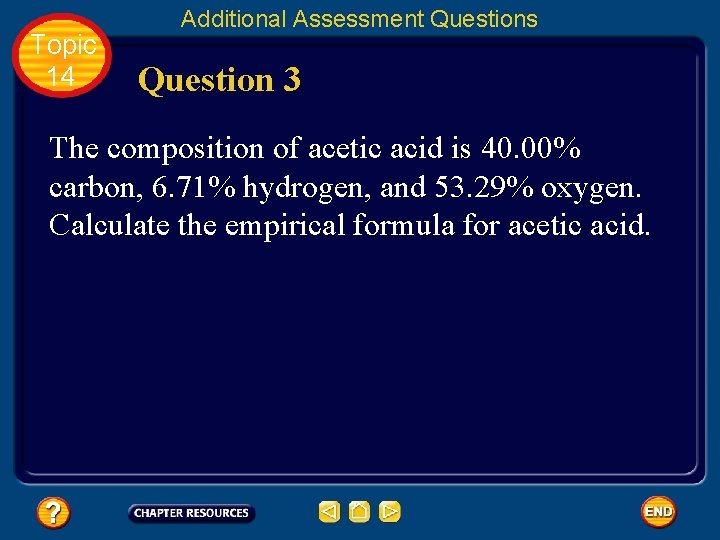Topic 14 Additional Assessment Questions Question 3 The composition of acetic acid is 40. 00% carbon, 6. 71% hydrogen, and 53. 29% oxygen. Calculate the empirical formula for acetic acid.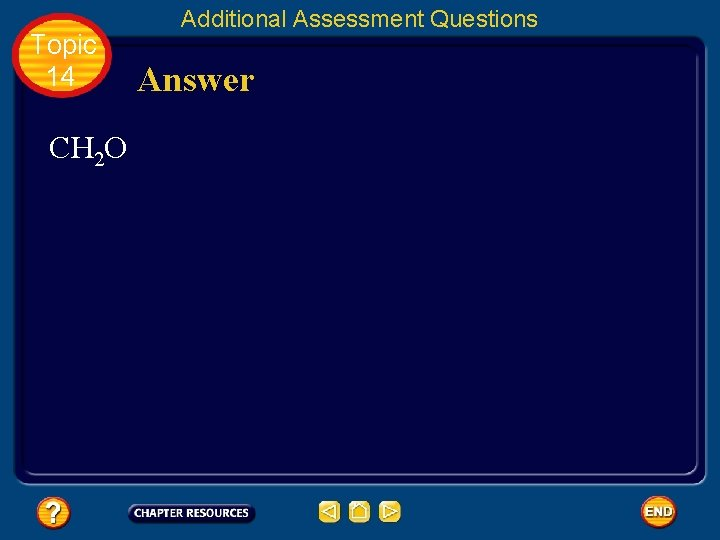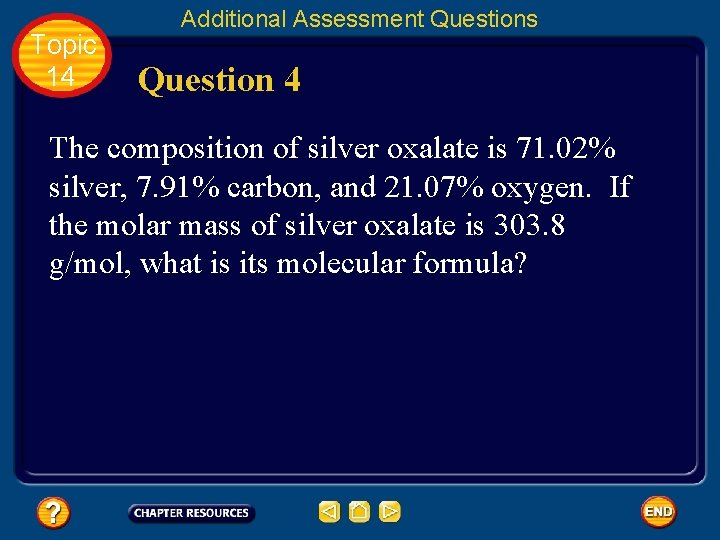Topic 14 Additional Assessment Questions Question 4 The composition of silver oxalate is 71. 02% silver, 7. 91% carbon, and 21. 07% oxygen. If the molar mass of silver oxalate is 303. 8 g/mol, what is its molecular formula?Topic 14 Additional Assessment Questions Answer Ag 2 C 2 O 4Help To advance to the next item or next page click on any of the following keys: mouse, space bar, enter, down or forward arrow. Click on this icon to return to the table of contents Click on this icon to return to the previous slide Click on this icon to move to the next slide Click on this icon to open the resources file. Click on this icon to go to the end of the presentation.End of Topic Summary File